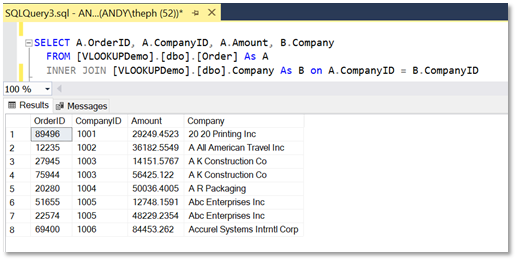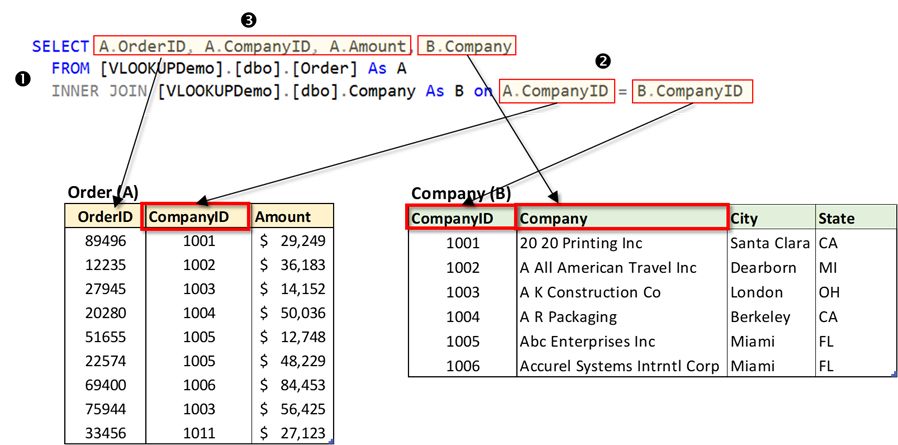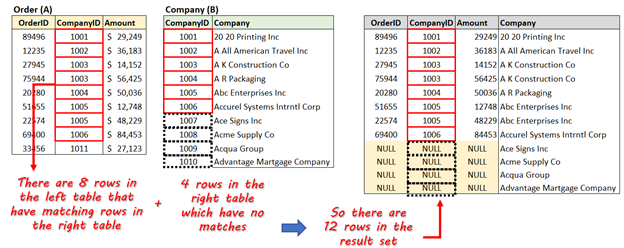• The Excel and SQL Server experts blog
• # What is the equivalent to VLOOKUP in SQL?

Andy McDonald - Jul 14, 2022

In this article, we’re going to show you how to perform the equivalent of Excel’s VLOOKUP in SQL.  This is the second of our articles aimed at teaching Excel users the basics of SQL. In our previous article “Introduction to SQL for Excel Users”, we introduced the JOIN statement, and here we’re going to expand on it further using a range of scenarios, with a particular emphasis on equivalent Excel usage.

## Excel VLOOKUP function: a recap

Ask a range of Excel users what their favorite or most used function is, and chances are that a lot of them will say “VLOOKUP”.  If you work in Excel on a day-to-day basis there’ll often be times when you need to wrangle data from a bunch of sources into a merged summary table for reporting or aggregation purposes.  The go-to function for this is VLOOKUP (or the MATCH / INDEX functions).

For those of you less familiar with VLOOKUP, here is a simple example to explain it.  There is a ‘Sales’ table which gives me sales amounts for companies, but it only references the CompanyID’s not the names.  The company name (and some other information) is stored in a separate table.  We therefore need to merge (or join) these tables.In cell D4, we can enter the following to populate the corresponding Company for CompanyID 1001:

``VLOOKUP(B4,\$G\$4:\$J\$13,2,FALSE)``

The formula says:

• lookup the value in cell B4
• in the range G4:J13, and …
• return the value in the second column of range G4:J13 where the value in B4 matches a value in the first column of range G4:J13I can add a similar formula in cell E4 and then copy it down to populate the table:

``VLOOKUP(B4,\$G\$4:\$J\$13,3,FALSE)``TIP: Microsoft introduced XLOOKUP in late 2019 as the replacement for VLOOKUP.  It adds a couple of cool new features and addresses some limitations in VLOOKUP.  Check out our article here to learn more about XLOOKUP.

## SQL Join Overview

In the introductory article, we showed you how to use the JOIN clause in a simple SQL query to get data from two related tables.  In this article, we’re going to look at a wider range of scenarios and introduce some variations of the simple JOIN clause.  Specifically, we’re going to show the equivalent of Excel’s VLOOKUP in SQL.

Before we continue, let’s just remind ourselves why we need to use the JOIN clause so often in SQL to merge data from different tables.  Why can’t the data be stored together in one table?  The reason is that for large databases, it is computationally inefficient to search ‘wide’ tables (ie tables with lots of columns).  Instead, it is much better to structure the data in multiple related tables.  This is called ‘data partitioning’ (specifically vertical partitioning) and is best understood with the simple example below.

The table below shows some information about some sales orders.  It provides lots of useful information, and we would be able to draw some interesting reports from it, such as ‘Sales Totals per Sales Rep’ or ‘Sales Totals per Region’.  However, if there were hundreds of thousands of rows, then it becomes inefficient to query.   It would be much better to partition this one big table into smaller tables and relate them to each other with ID columns.Something like this would work:Of course, now that the data is in different tables, to perform any meaningful queries, we’re going to need to include the JOIN clause in most of our queries.  Almost all databases partition the data in this way, so learning how to join tables is very important.

To summarize: a JOIN clause is used in SQL to combine rows from two or more tables, based on a related column between them.

## How to use the JOIN clause to mimic VLOOKUP in SQL

Ok, now that we have re-capped everything, let’s look at how to use the JOIN clause to mimic the behavior of VLOOKUP in SQL – if you want to follow along, the SQL database is here. We want to query the Order table and to include the Company name instead of just the CompanyID.  Let’s look at the query and the results and then examine how it works.

``````SELECT A.OrderID, A.CompanyID, A.Amount, B.Company
FROM [VLOOKUPDemo].[dbo].[Order] As A
INNER JOIN [VLOOKUPDemo].[dbo].Company As B on A.CompanyID = B.CompanyID``````The INNER JOIN works using the fact that there is a common column between the 2 tables we want to join – in our example it is the CompanyID column.  The query therefore basically says “return the columns specified (OrderID, CompanyID, Amount, Company) from the two related tables where values in the CompanyID columns are equal”.  Here is more of a breakdown:

• The INNER JOIN statement is added after the first related table (the “left” table) is specified – the Order table. Note that we have added a ‘table alias’, A, for the order table; this just makes it easier to read the query when we have long table names.  Next, we need to specify the table on the other side of the join (the “right” table) – the Company table.  Again, we give it an alias, B.
• Now we need to specify the columns we want to match values on – the CompanyID column in each table. Note that we need to qualify the column names with the respective table alias names.
• Note that we’ve prefixed the column names with the table alias names – we do this because often columns will have the same name (“Name” is commonly used for columns, for example) and we need to avoid ambiguity.The eagle-eyed of you may have noticed something about the results returned by our INNER JOIN query – the Order table has 9 records in it, but the results from our JOIN query only show 8 records.Why is there one missing?  The answer lies in how the JOIN clause handles the situations when there are no matching rows in one or other of the joined tables.  For example, if we were to use the same data and use a VLOOKUP function in Excel, we would have got the following results:We’ll therefore need to look at a variation of the normal JOIN clause to handle this situation.  In the next section, therefore, we look at the other types of SQL JOINS.

## Other types of SQL Joins

When we include a JOIN clause in a SQL query, it is normally an ‘inner’ join, but there are other types, which we’re going to look at now.  First, let’s summarise the INNER JOIN.

### INNER JOIN

The INNER JOIN will only return results where there are matching values for our common column in BOTH the left-hand and right-hand tables.

``````SELECT A.OrderID, A.CompanyID, A.Amount, B.Company
FROM [VLOOKUPDemo].[dbo].[Order] As A
INNER JOIN [VLOOKUPDemo].[dbo].Company As B on A.CompanyID = B.CompanyID``````### LEFT OUTER JOIN

The LEFT OUTER JOIN will return ALL of the rows in the left-hand table.  If there is a row in the left-hand table which has no match in the right-hand table, then the row is included in our result set, with a NULL returned for the lookup value.   The behavior of the LEFT OUTER JOIN mimics how the VLOOKUP function in Excel returns results.  In our example below, we can see that we have a missing company in our Company table, so we’d need to fix that.  If we had used the INNER JOIN query, we would not have picked up the missing company issue.

``````SELECT A.OrderID, A.CompanyID, A.Amount, B.Company FROM [VLOOKUPDemo].[dbo].[Order] As A
LEFT OUTER JOIN [VLOOKUPDemo].[dbo].Company As B on A.CompanyID = B.CompanyID``````### RIGHT OUTER JOIN

The RIGHT OUTER JOIN will return rows in the left-hand table where there are matching values for our common column in BOTH the left-hand and right-hand tables table.  It will also return the remaining non-matching rows from the right-hand table, with NULLS displayed for the OrderID, CompanyID, and Amount columns.

``SELECT A.OrderID, A.CompanyID, A.Amount, B.Company FROM [VLOOKUPDemo].[dbo].[Order] As A RIGHT OUTER JOIN [VLOOKUPDemo].[dbo].Company As B on A.CompanyID = B.CompanyID``### FULL OUTER JOIN

The FULL OUTER JOIN returns ALL the rows from both tables, with NULLS displayed for non-matching records.

``SELECT A.OrderID, A.CompanyID, A.Amount, B.Company FROM [VLOOKUPDemo].[dbo].[Order] As A FULL OUTER JOIN [VLOOKUPDemo].[dbo].Company As B on A.CompanyID = B.CompanyID     ``## Summary

In this article, we’ve explained why most databases are made up of multiple related tables.  As Excel users, we know all about handling data in different tables or sheets, and we have the VLOOKUP (and HLOOKUP) functions to help us lookup matching data.

To get the same behavior of VLOOKUP in SQL, we need to get data from multiple SQL tables, and we do this using the JOIN statement.  As we’ve seen, it’s not enough to simply specify the tables we want to join (and which column needs to be used to match data) – we also need to specify what to do with the rows where there is no matching data.

We looked at 4 different types of join clauses. As we’ve seen, it’s important to use the correct one to make sure the results you get are complete.  In terms of a direct comparison to the VLOOKUP function in Excel, the LEFT OUTER JOIN type is the equivalent in SQL.

Do you want to view and update SQL data from within Excel?  Try the SQL Spreads Excel Add-In today.

*This article was originally published on September 11, 2021 and was updated on July 14, 2022 to include some updated screenshots.Gyan » 18. Dec, 2021

Amazing teaching… Great Job keep it up!JEFF » 19. Jan, 2022

Is there any real vlookup way in SQL if table B is not the unique key?
EXAMPLE:
table A is 20 rows with unique key
table B is 30 rows with non-unique key

IN vlookup:
result:
20 rows (duplicate result depend on sorting of table B )

IN left join:
result:
may be 20+ rows

I want the result is 20 rows of table A.Andy McDonald » 21. Jan, 2022

Hi Jeff, can you share the example that you are looking at?Ty » 20. Jan, 2022

work for Mysql?Andy McDonald » 23. Jan, 2022

Hi Ty, yes, all the join queries presented here will work, with the exception of the FULL OUTER join. MySQL doesn’t support full outer join out of the box, but you can mimic one using a combination of LEFT JOIN ad RIGHT JOIN. Something like the below should work:

SELECT A.OrderID, A.CompanyID, A.Amount, B.Company
FROM vlookup_demo.order As A
LEFT JOIN vlookup_demo.company As B ON A.CompanyID = B.CompanyID
UNION
SELECT A.OrderID, A.CompanyID, A.Amount, B.Company
FROM vlookup_demo.order As A
RIGHT JOIN vlookup_demo.company As B ON A.CompanyID = B.CompanyID;Subhash » 13. Apr, 2022

Thank you Sir. The article helped me understand the exact use of different types of joins.David Myers » 15. Jun, 2022

Andy: Do you have the demo database for this tutorial that you can make available? If you’ve linked to it in this tutorial somewhere, I can’t seem to find it. I’m a SQL novice (but an experienced Excel user) and I’d like to follow along with this tutorial by actually trying the queries you demonstrate here. Thanks!Andy McDonald » 15. Jun, 2022

Hi David, here is the link to the demo database; I’ve also added it to the article.